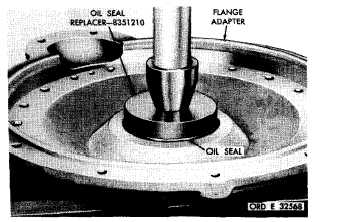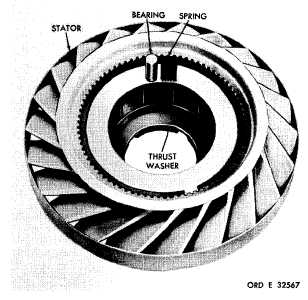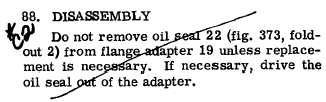S e c t i o n    V .    F L A N G E    A D A P T E R    A S S E M B L Y  R E B U I LD
Custom Search*C2 C H A P    5,   SEC  V F L A N G E     A D A P T E R     R E B U I LD PAR   6 - 91 (4)   Install   thrust   washer   100   and   front- washer   assembly   81.   Install   retaining   ring   80. ( 5 )    I n s t a l l    t h r u s t    w a s h e r    1 0 3    i n t o    t he s t a t o r    a s s e m b l y. (6)   Install   thrust   bearing   assembly   102 o n t o    f r e e w h e e l    r a c e    1 0 1 ,    u s i n g    o i l - s o l u b l e grease   to   retain   it. (7)   Using   a   heavy   oil-soluble   grease   to r e t a i n    t h e m ,    p o s i t i o n    t h e    1 2    b e a r i n g s    a n d springs   in   the   12   stator   cam   pockets.   Fig.   148 shows   the   location   of   the   bearings   and   springs i n    r e s p e c t    t o    t h e    s t a t o r    a s s e m b l y .    I n s t a l l    a spring   into   the   deep   end   of   the   cam   pocket. Place   a   bearing   in   the   shallow   end   of   the   cam p o c k e t    b e s i d e    t h e    s p r i n g .    I n s t a l l    r e m a i n i ng 11   bearings   and   springs   in   the   same   manner. (8)   Install   freewheel   race   101   (fig.   373, fold-out   2)   with   bearing   102   into   the   stator   as- s e m b l y . Figure 148. Torque converter stator S e c t i o n     V .     F L A N G E     A D A P T E R     A S S E M B L Y — R E B U I LD 8 7 .     D E S C R I P T I O N R e f e r    t o    p a r .    8    f o r    t h e    d e s c r i p t i o n    o f    t h e f l a n g e    a d a p t e r    a s s e m b l y . 8 9 .    C L E A N I NG Refer   to   par.   71   for   cleaning   recommend- a t i o n s . 9 0 .    I N S P E C T I O N    A N D    R E P A IR R e f e r    t o    p a r .    7 2    f o r    g e n e r a l    i n s p e c t i on and    repair    recommendations.    Repair    and    re- b u i l d    p o i n t s    o f    m e a s u r e m e n t    f o r    f i t s ,    c l e a r- ances   and   wear   limits   are   indicated   by   small letters   in   fig.   373,   fold-out   2.   Refer   to   par. 236   for   wear   limits   information. 1 0 8 9 1 .    A S S E M B L Y .   If   the   oil   seal   was   removed   from   the flange   adapter,   install   a   new   replacement   using oil   seal   replacer   8351210   (fig.   149). .   Install   oil   seal,   lip   side   down,   press- i n g    t h e    s e a l    u n t i l    i t    i s    s e a t e d    a g a i n s t    i t s shoulder   in   the   adapter   (fig.   149). Figure 149. Installing oil seal in flange adapterIntegrated Publishing, Inc. - A (SDVOSB) Service Disabled Veteran Owned Small Business